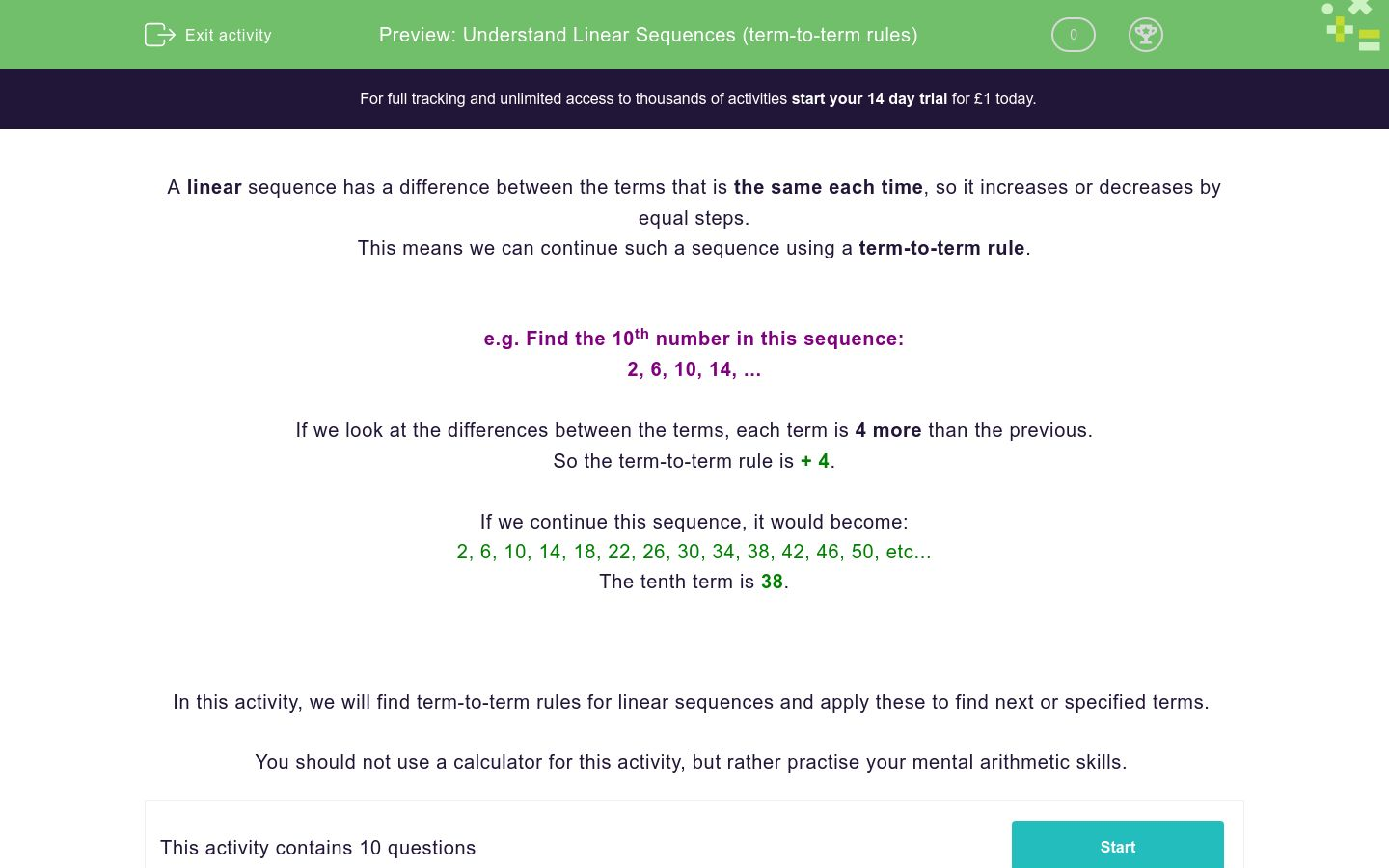# Understand Linear Sequences (Term to Term)

In this worksheet, student practise generating sequences using term to term rules.Key stage:  KS 4

GCSE Subjects:   Maths

GCSE Boards:   AQA, Eduqas, Pearson Edexcel, OCR

Curriculum topic:   Algebra

Curriculum subtopic:   Sequences

Difficulty level:### QUESTION 1 of 10

A linear sequence is a sequence that has a difference between the terms that is the same each time.

This means we can continue by using a term-to-term rule.

Example: Find the 10th number in this sequence.

2, 6, 10, 14...

If we look at the differences, each term is 4 more than the next, so the term to term rule is +4.

If we continue the sequence we get 2, 6, 10, 14, 18, 22, 26, 30, 34, 38, 42, 46, 50

The tenth term is 38

A linear sequence has a common ...

What is the rule for this sequence?

6, 11, 16, 21...

What is the 11th term in this sequence?

3, 7, 11, 15

James says that the 20th number in the sequence 2, 5, 8, 11 will be double the 10th.

Is he correct?

Yes

No

Which is the first number in the sequence 1, 5, 9 that will be greater than 50?

How many positive numbers are in the sequence 25, 23, 21....

Match the sequence with its next two numbers.

## Column B

12, 15, 18
0, -5
1, 2, 3, 4
21, 24
20, 15, 10 ,5
1, -4
16, 11, 6
5, 6

Match the sequence with the correct 12th term.

## Column B

3, 5, 7, 9...
1
10, 13, 16, 19...
43
11, 8, 5, 2...
25
1, 1, 1, 1...
-22

Which of the following numbers will appear in the sequence 3, 8, 13, 18...

23

38

0

-3

25

10000457893

Which of the following numbers will appear in the sequence...

22, 18, 14 ...

4

-6

-5

0

26

10

• Question 1

A linear sequence has a common ...

difference
EDDIE SAYS
The proper name for how much the sequence increase is the common difference.
• Question 2

What is the rule for this sequence?

6, 11, 16, 21...

+5
5
EDDIE SAYS
The easiest way to find the rule is to subtract one number from the number following it. For example 21 - 16 = + 5
• Question 3

What is the 11th term in this sequence?

3, 7, 11, 15

41
EDDIE SAYS
All we have to do here is find the common difference (7-3 = 4) and keep going with the sequence until we have 11 numbers.
• Question 4

James says that the 20th number in the sequence 2, 5, 8, 11 will be double the 10th.

Is he correct?

No
EDDIE SAYS
This is a really common mistake to make. The only way you can do this is to find both the 10th and 20th terms and then compare them. The 10th term is 29 The 20th term is 59
• Question 5

Which is the first number in the sequence 1, 5, 9 that will be greater than 50?

53
EDDIE SAYS
We can see that the rule for this sequence is +4. If we continue the sequence we will get ... 49, 53, 57.... We can see from here what the first number bigger than 50 is.
• Question 6

How many positive numbers are in the sequence 25, 23, 21....

13
EDDIE SAYS
If we continue this sequence, we will eventually drop below zero. We can see from this list how many numbers there are before that happens.
• Question 7

Match the sequence with its next two numbers.

## Column B

12, 15, 18
21, 24
1, 2, 3, 4
5, 6
20, 15, 10 ,5
0, -5
16, 11, 6
1, -4
EDDIE SAYS
A nice easy one, just find the term to term rule and keep going.
• Question 8

Match the sequence with the correct 12th term.

## Column B

3, 5, 7, 9...
25
10, 13, 16, 19...
43
11, 8, 5, 2...
-22
1, 1, 1, 1...
1
EDDIE SAYS
All we have to do here is to continue each sequence till there are 12 numbers. For example 3, 5, 7, 9, 11, 13, 15, 17, 19, 21, 23, 25
• Question 9

Which of the following numbers will appear in the sequence 3, 8, 13, 18...

23
38
10000457893
EDDIE SAYS
If we look at the sequence, we can see that every number has either a 3 or an 8 at the end of it. Sequences can't go down so all the negative numbers are out.
• Question 10

Which of the following numbers will appear in the sequence...

22, 18, 14 ...

4
-6
10
EDDIE SAYS
If you continue this sequence by taking 4 off each time, you\'ll spot the right and wrong ones here.
---- OR ----

Sign up for a £1 trial so you can track and measure your child's progress on this activity.

### What is EdPlace?

We're your National Curriculum aligned online education content provider helping each child succeed in English, maths and science from year 1 to GCSE. With an EdPlace account you’ll be able to track and measure progress, helping each child achieve their best. We build confidence and attainment by personalising each child’s learning at a level that suits them.

Get started